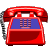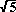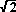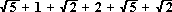# Phone Number "Lengths"

Everybody knows that, in general, a telephone number consists of seven digits, right? So if we asked a rather innocent question: "How long is your phone number?", no one would think very much was odd if the answer were "7 digits long", correct? But what if you heard the response of 10.30 from one individual and 12.64 from another individual? You knew the respective phone numbers were 278-6490 and 324-0391; so what could those people be doing?

Since we're talking about phone numbers, let's look at the typical arrangement of the digits on the phone itself. It should resemble something like the grid pictured below.

 1 2 3 4 5 6 7 8 9 0

Recall how you mark, or "dial", a number on such a phone as this. For the first example cited above, you would press the 2, then move your finger directly to the 7, then to the 8, and so on. But as you transferred your finger from the 2 to the 7, you actually traced the hypotenuse of a right triangle that had legs of length 2 units and 1 unit. Enter the Pythagorean Theorem: the square of the hypotenuse is equal to the sum of the squares of the other two sides (legs). In math, we would summarize that as follows:

(hypotenuse)2 = (leg1)2 + (leg2)2
So our distance from 2 to 7 would be computed in this way:

(hypotenuse)2 = 22 + 12 = 4 + 1 = 5

hypotenuse =.

Of course, not all cases need the Pythagorean Theorem, as the movement from 7 to 8 shows; it is merely the distance of 1 (unit). The rest of our phone number works out as follows:

8 to 6 is; 6 to 4 is 2; 4 to 9 is; and 9 to 0 is.

This makes a grand total ofUsing our calculator gives 10.30 (approximately). Now you do the other phone number -- for homework!!!